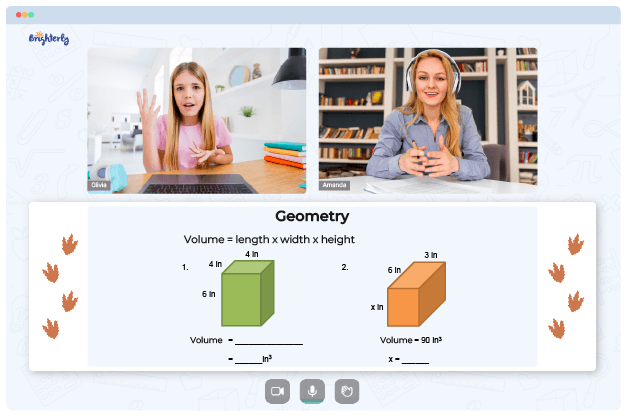# Measuring Angles With A Protractor Worksheets

A protractor is a transparent plastic tool used to measure angles from 0° to 180°—the two sets of numbers on a protractor increase in opposite directions. Kids can get an accurate angle by placing the protractor’s center at a point where they want to measure.

Continue reading to see why measuring angles with a protractor worksheet is essential to every child.

## Importance of Measuring Angles with a Protractor

Knowing how to measure an angle is a crucial skill for every child who wants to understand geometry. Before classifying an angle as obtuse, acute, or right, kids need to know how a protractor works.

In careers like surveying, construction, carpentry, engineering, cartography, etc., accurate measurement of angles is critical. Knowledge of angles is also helpful in astrology to compute the location and movement of stars. This way, one can determine the stars’ distance and estimate their relative sizes.

Math for Kids

Is Your Child Struggling With Math?
1:1 Online Math Tutoring## How Tutors at Brighterly Can Use Measuring Angles with Protractor Worksheets

Brighterly’s tutors can provide kids with a chance to understand math better. The tutors can also help bridge the knowledge gap caused by a lack of foundational math knowledge.

Tutors understand that handling the topic of protractors and angles can be challenging for kids and adults alike. So, they recommend using measure angles with a protractor worksheet. But if you have never utilized a protractor to measure angles on a diagram or drawing, you are not alone.### Measuring Angles With A Protractor Worksheet PDF

Measuring Angles With A Protractor Worksheet### Measuring Angles With A Protractor Worksheet PDF

Measuring Angles With Protractor Worksheets### Measuring Angles With A Protractor Worksheet PDF

Measure Angles With A Protractor Worksheet### Measuring Angles With A Protractor Worksheet PDF

Measuring Angles On A Protractor Worksheet

Tutors use a measuring angles on a protractor worksheet to explain to kids how to use the tool: set the protractor’s middle point to measure an angle accurately. Worksheets usually contain pictorial representations of protractors; so, kids can see where the vertical line from the 90° mark meets its baseline. It is necessary to match one side of the angle with the protractor baseline.

Activities in worksheets are usually hand-on; so, when kids figure out where the opposite side of an angle meets the numbered scale on the protractor’s outer edge, they are to take a reading of the angle in degrees. Kids can also get measuring angles with a protractor worksheet in PDF from their tutors to practice at their convenience.

### More Angles Worksheets

Need help with Geometry?• Start lessons with an online tutor.

Is your child finding it challenging to master geometry concepts? An online tutor could be the solution.